# 1496 is a Square Pyramidal Number

### Visualize 1496 Blocks:

I hoped to make a graphic illustrating that 1496 is the 16th square pyramidal number. I am thrilled that I succeeded!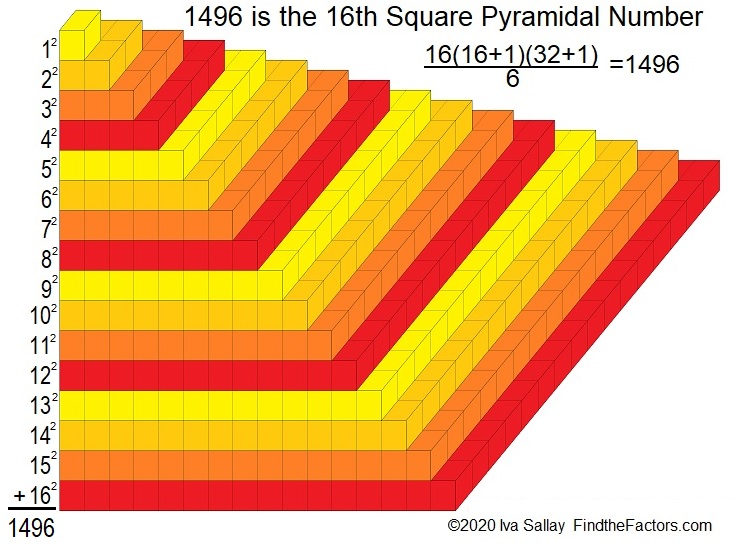### Factors of 1496:

Knowing the multiplication table and some divisibility tricks helped me find some of 1496’s factors:
96 is 8 × 12, and 4 is even, so 1496 is divisible by 8.
1 – 4 + 9 – 6 = 0, so 1496 is divisible by 11.

• 1496 is a composite number.
• Prime factorization: 1496 = 2 × 2 × 2 × 11 × 17, which can be written 1496 = 2³ × 11 × 17
• 1496 has at least one exponent greater than 1 in its prime factorization so √1496 can be simplified. Taking the factor pair from the factor pair table below with the largest square number factor, we get √1496 = (√4)(√374) = 2√374
• The exponents in the prime factorization are 3,1 and 1. Adding one to each exponent and multiplying we get (3 + 1)(1 + 1) (1 + 1) = 4 × 2 × 2 = 16. Therefore 1496 has exactly 16 factors.
• The factors of 1496 are outlined with their factor pair partners in the graphic below.### Another fact about the number 1496:

1496 is the hypotenuse of a Pythagorean triple:
704-1320-1496, which is (8-15-17) times 88.

# 1240 is a Square Pyramidal Number

1240 is the 15th square pyramidal number because
1² + 2² + 3² + 4² + 5² + 6² + 7² + 8² + 9² + 10² + 11² + 12² + 13² + 14² + 15² = 1240

We can know that 1240 is the 15th square pyramidal number because
15(15 + 1)(2·15 + 1)/6
= 15(16)(31)/6
= (5)(8)(31)
= (40)(31)
= 1240Here are some more facts about the number 1240:

• 1240 is a composite number.
• Prime factorization: 1240 = 2 × 2 × 2 × 5 × 31, which can be written 1240 = 2³ × 5 × 31
• The exponents in the prime factorization are 3, 1, and 1. Adding one to each and multiplying we get (3 + 1)(1 + 1)(1 + 1) = 4 × 2 × 2 = 16. Therefore 1240 has exactly 16 factors.
• Factors of 1240: 1, 2, 4, 5, 8, 10, 20, 31, 40, 62, 124, 155, 248, 310, 620, 1240
• Factor pairs: 1240 = 1 × 1240, 2 × 620, 4 × 310, 5 × 248, 8 × 155, 10 × 124, 20 × 62, or 31 × 40
• Taking the factor pair with the largest square number factor, we get √1240 = (√4)(√310) = 2√310 ≈ 35.21363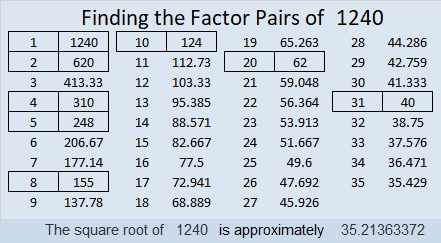1240 is the hypotenuse of a Pythagorean triple:
744-992-1240 which is (3-4-5) times 248

# 650 is the sum of all the clues in this Level 1 puzzle

1² + 2² + 3² + 4² + 5² + 6² +7² + 8² + 9² +10² + 11² + 12²  = 650

Thus 650 is the 12th square pyramidal number and can be calculated using 12(12 +1)(2⋅12 + 1)/6.

If you add up all the clues in today’s Find the Factors puzzle, you will get the number 650. However, if you print the puzzle from the excel file, one of the clues is missing because it isn’t needed to find the solution.

650 is the hypotenuse of seven Pythagorean triples!

• 72-646-650
• 160-630-650
• 182-624-650
• 250-600-650
• 330-560-650
• 390-520-650
• 408-506-650

Can you find the greatest common factor of each triple? Each greatest common factor will be one of the factors of 650 listed below the puzzle.

650 is the hypotenuse of so many Pythagorean triples because it is divisible by 5, 13, 25, 65, and 325 which are also hypotenuses of triples. The smallest three numbers to be the hypotenuses of at least 7 triples are 325, 425, and 650.

Since 25 x 26 = 650, we know that (25-1)(26 + 1) = 650 – 2. Thus 24 x 27 = 648.Print the puzzles or type the solution on this excel file: 12 Factors 2015-10-19

—————————————————————————————————

• 650 is a composite number.
• Prime factorization: 650 = 2 x 5 x 5 x 13, which can be written 650 = 2 x (5^2) x 13
• The exponents in the prime factorization are 1, 2, and 1. Adding one to each and multiplying we get (1 + 1)(2 + 1)(1 + 1) = 2 x 3 x 2 = 12. Therefore 650 has exactly 12 factors.
• Factors of 650: 1, 2, 5, 10, 13, 25, 26, 50, 65, 130, 325, 650
• Factor pairs: 650 = 1 x 650, 2 x 325, 5 x 130, 10 x 65, 13 x 50, or 25 x 26
• Taking the factor pair with the largest square number factor, we get √650 = (√25)(√26) = 5√26 ≈ 25.495098.—————————————————————————————————

# 506 and Level 5

506 is divisible by 11 because 5 + 6 – 0 = 11, and 11 obviously is divisible by 11.

506 is the 11th square pyramidal number because it is the sum of the first eleven square numbers.

Thus 1 + 4 + 9 + 16 + 25 + 36 + 49 + 64 + 81 + 100 + 121 = 506.That was predictable because 506 = (11 x 12 x 23)/6 and 12 = 11 + 1 and 23 = 2(11) + 1.

Since 506 = 22 x 23, it is the sum of the first 22 even numbers which also happens to be exactly two times the 22nd triangular number, 253.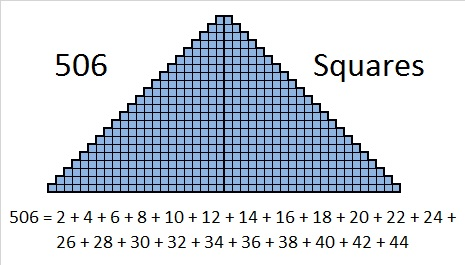Now here’s a Level 5 puzzle for you to try: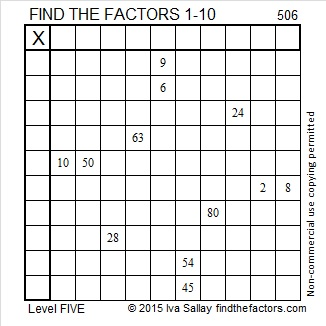Print the puzzles or type the solution on this excel file: 10 Factors 2015-05-25

—————————————————————————————————

• 506 is a composite number.
• Prime factorization: 506 = 2 x 11 x 23
• The exponents in the prime factorization are 1, 1, and 1. Adding one to each and multiplying we get (1 + 1)(1 + 1)(1 + 1) = 2 x 2 x 2 = 8. Therefore 506 has exactly 8 factors.
• Factors of 506: 1, 2, 11, 22, 23, 46, 253, 506
• Factor pairs: 506 = 1 x 506, 2 x 253, 11 x 46, or 22 x 33
• 506 has no square factors that allow its square root to be simplified. √506 ≈ 22.49444—————————————————————————————————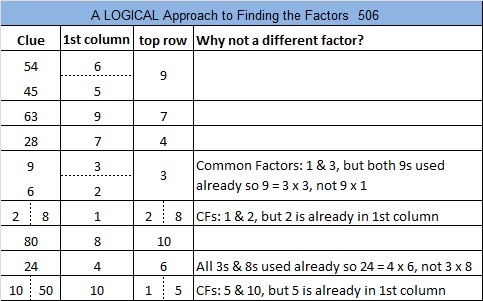# 385 Is a Square Pyramidal Number

385 is the sum of the squares of the first ten counting numbers. Let me demonstrate what that means. All of the following are square pyramidal numbers:

• 1² = 1
• 1² + 2² = 5
• 1² + 2² + 3² = 14
• 1² + 2² + 3² + 4² = 30
• 1² + 2² + 3² + 4² + 5² = 55
• 1² + 2² + 3² + 4² + 5² + 6² = 91
• 1² + 2² + 3² + 4² + 5² + 6² + 7² = 140
• 1² + 2² + 3² + 4² + 5² + 6² + 7² + 8² = 204
• 1² + 2² + 3² + 4² + 5² + 6² + 7² + 8² + 9² = 285
• 1² + 2² + 3² + 4² + 5² + 6² + 7² + 8² + 9² + 10² = 385

We could have found the total, 385, much more quickly by putting the number ten in for “n” in this formulaWhich means the sum of the first ten square counting numbers = (10 x 11 x 21)/6 = 5 x 11 x 7 = 385. (Notice its prime factorization!)

Here’s a video explanation: